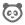cancel
Showing results for
Did you mean:

# New Quizzes: Multiple Answers in a Formula Question

In the Canvas Community Ideas space, you can share, converse, and rate idea conversations related to software improvements to Canvas products.

# New Quizzes: Multiple Answers in a Formula Question

This idea is based on extending the Formula Question type to allow for multiple answers to be entered by students.

Currently, the Formula question type is fine for simple calculated answer questions that only ask for one final response from students. We have several instructors that would like to generate data based on ranges, but also to ask for several answers based on that generated data.

One example of this would be a financial statement or budget sheet. Instructors and Designers would like to generate random data elements for some parts of these sheets, but ask for multiple answers from the same set of generated data such as the following list of questions:

"What is the Inventory Turnover? What is the AR Turnover? What is the AP Turnover? What is the Inventory Period? What is the AR Period? What is the Operating Cycle? What is the Cash Cycle? What is Net Working Capital for 2013?"

Each answer is based on the same data, but uses different formulas to determine the correct result.

Community Member

Is this really not an already implemented feature?  So if I write up a forumula problem I can't ask "What is the first derivative" followed by "what is the second derivative?"

Navigator

marasco.physics@gmail.com, the last time I checked (September 15), no this is not currently an option for a formula type question.

However, if you create Multiple Dropdown or Multiple Fill in the blank questions, you can do it, but there are problems with those and they're not formula type questions.

You may want to read some of these discussions for additional background.

Community Member

I only get to vote for this once?!  This is really an essential capability for good science questions -- and apparently in other fields as well.  Being able to describe a rich set of given information, requiring students to decide what is important, and then calculate multiple relevant answers is a BIG pedagogical advantage.  Otherwise the tendency is for "hit and run" assessment which is quite artificial.  Ask your friends to vote for this.

Community Member

Folks, it truly boggles my mind that a stats instructor cannot generate a set of random numbers, and then ask students to compute both the mean and the standard deviation.

How is this not broken?

Community Member

I have found many times that two answers would be way better for math and physics formula questions.

Community Member

I would like this feature added. I would like students to be able to give just one answer but that answer is checked against more than one formula. Could help in a situation where students could get specific feedback based on their answer.  Often in Physics or Maths, one small mistake can be made (for example, not converting a value from km to m) that would lead to the wrong answer. In that situation, students can be given partial marks.

Community Member

As others have stated, please add this feature.  Perhaps a feasible way of implementing this would be that any variables created within a question group would remain constant for all questions in that group.Instructure

Hello, Canvas Family!

Multi-variable formulas are a bit more challenging to implement. We will want to look at the best means to implement this from an item authoring and delivery process. We are exploring a series of extensive improvements to the quizzes toolset. I know we won't be able to deploy this idea in the next six months; however, be assured we've listened and will add this to our set of ideas for improvements to quizzes.

Kind regards,

Jason

Navigator

Thank you for the exciting news Jason.

Community members can follow the larger project at Modern Quizzing Engine

Community Member

Is there any progress on this?  It's very frustrating to only be able to ask one question from a prompt.  I can't even ask something as simple as "given the following randomized formula, please find the first and second derivatives."